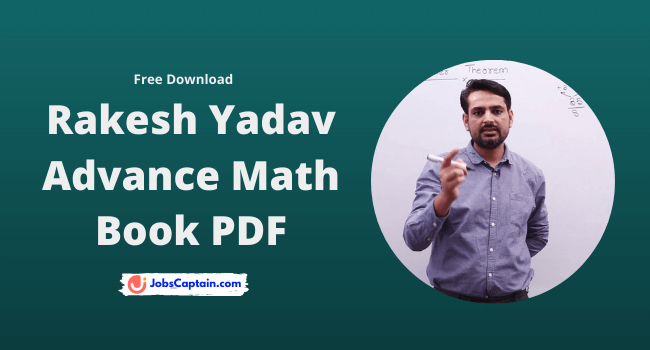By | April 13, 2022Rakesh Yadav Sir is one of the most renounced faculty of Maths in India for all kinds of competitive Exams and is teaching Mathematics.

In this post, we are going to share with you Advance Math Book PDF of Rakesh Yadav sir. This math book is very useful to you in all competitive exams such as SSC CGL, Railway Group D, Defence and Banking Exam (IBPS-SBI).

From this book, you will learn 20 important topics of Math. Each and every chapter contains practice questions. By practising questions you will be able to sharpen your math question solving skills.

## Book Content

Following Advance Maths topics covered in this book:

1. Indices and Surds
2. Linear equation in two variable
3. Graphic representation of straight lines
4. Coordinate geometry
5. Polynomials
6. Mensuration – I (Area and Perimeter (2D)
7. Mensuration – II (Area and Volume (3D)
8. Algebraic Identities
9. Line
10. Triangles
12. Center of Triangles
13. Triangles, Congruence, and Similarity
14. Circles, Chords, and Tangents
15. Trigonometric ratios
16. Trigonometric Identities
17. Maximum and Minimum value of Trigonometry functions
18. Trigonometry, Circular Measurement of Angles
19. Height & Distance

• Very useful book according to the SSC CGL point of view
• Excellent concept & solution to all the questions.
• Handy book for quick revision of important Math Topics like Geometry, Algebra, Trigonometry, Menstruation, Coordinate Geometry etc.
• Easy solving methods and easy explanation

Furthermore, if you have any doubts about math subject then do comment below. Our team will try to help you.